Functions and CALL Routines

# BLACKCLPRC Function

Calculates call prices for European options on futures, based on the Black model.
 Category: Financial

## Syntax

 BLACKCLPRC(E, t, F, r, sigma)

### Arguments

E

is a non-missing, positive value that specifies exercise price.

 Requirement: Specify E and F in the same units.
t

is a non-missing value that specifies time to maturity.

F

is a non-missing, positive value that specifies future price.

 Requirement: Specify F and E in the same units.
r

is a non-missing, positive fraction that specifies the risk-free interest rate between the present time and t.

 Requirement: Specify a value for r for the same time period as the unit of t.
sigma

is a non-missing, positive fraction that specifies the volatility (the square root of the variance of r).

 Requirement: Specify a value for sigma for the same time period as the unit of t.

The BLACKCLPRC function calculates call prices for European options on futures, based on the Black model. The function is based on the following relationship:
where

 F specifies future price. N specifies the cumulative normal density function. E specifies the exercise price of the option. r specifies the risk-free interest rate for period t. t specifies the time to expiration.
where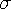specifies the volatility of the underlying asset.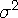specifies the variance of the rate of return.

For the special case of t=0, the following equation is true:
For information about the basics of pricing, see Using Pricing Functions.

The BLACKCLPRC function calculates call prices for European options on futures, based on the Black model. The BLACKPTPRC function calculates put prices for European options on futures, based on the Black model. These functions return a scalar value.

SAS Statements Results

`----+----1----+----2--`
```a=blackclprc(1000, .5, 950, 4, 2);
put a;```
`65.335687119`
```b=blackclprc(850, 2.5, 125, 3, 1);
put b;```
` 0.012649067`
```c=blackclprc(7500, .9, 950, 3, 2);
put c;```
`17.880939441`
```d=blackclprc(5000, -.5, 237, 3, 2);
put d;```
`           0`

 Function:Previous Page | Next Page | Top of Page